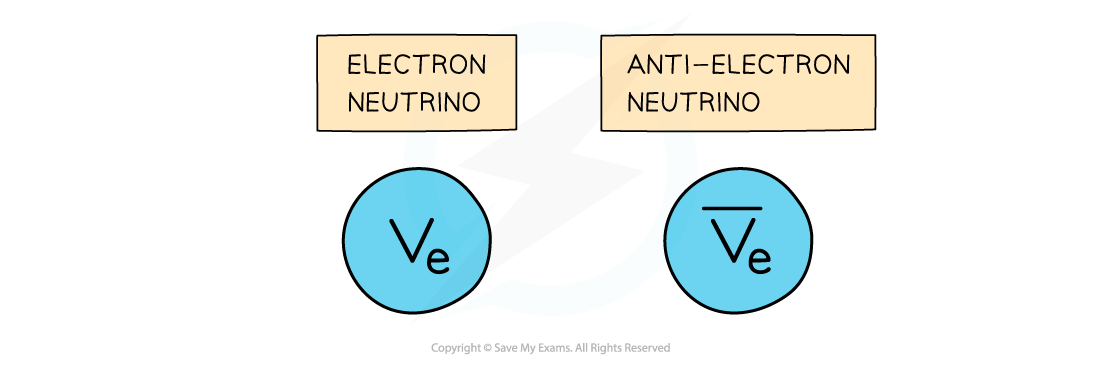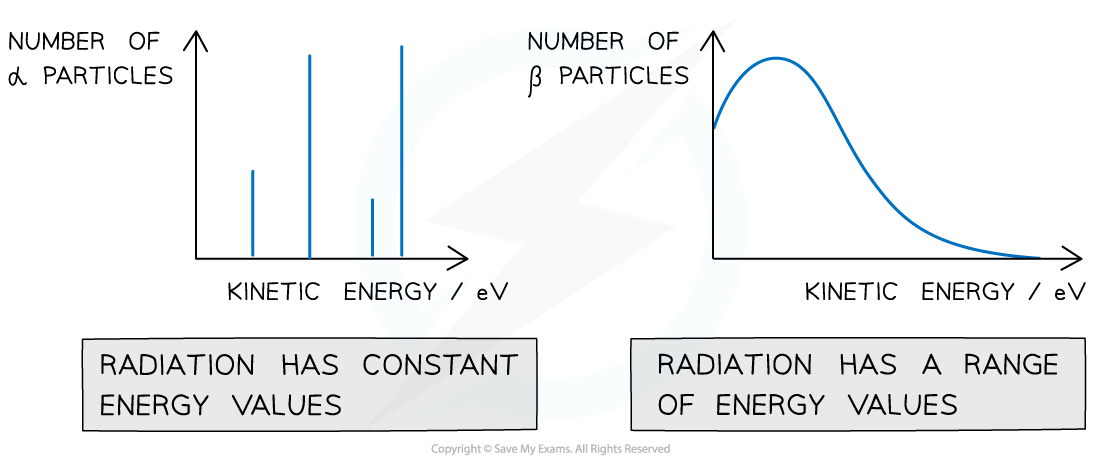# IB DP Physics: HL复习笔记12.2.5 The Neutrino

### The Neutrino

• An electron neutrino is a type of subatomic particle with no charge and negligible mass which is also emitted from the nucleus
• The anti-neutrino is the antiparticle of a neutrino
• Electron anti-neutrinos are produced during β– decay
• Electron neutrinos are produced during β+ decay• Although the neutrino has no charge and negligible mass, its existence was hypothesised to account for the conservation of energy in beta decay
• When the number of α particles is plotted against kinetic energy, there are clear spikes that appear on the graph
• This demonstrates that α-particles have discrete energies (only certain values)Alpha particles have discrete energy levels whilst beta particles have a continuous range of energies

• When the number of β particles is plotted against kinetic energy, the graph shows a curve
• This demonstrates that beta particles (electrons or positrons) have a continuous range of energies
• This is because the energy released in beta decay is shared between the beta particles (electrons or positrons) and neutrinos (or anti-neutrinos)
• This was one of the first clues of the neutrino’s existence
• The principle of conservation of momentum and energy applies in both alpha and beta emission

#### Exam Tip

One way to remember which particle decays into which depends on the type of beta emission, think of beta ‘plus’ as the ‘proton’ that turns into the neutron (plus an electron neutrino)